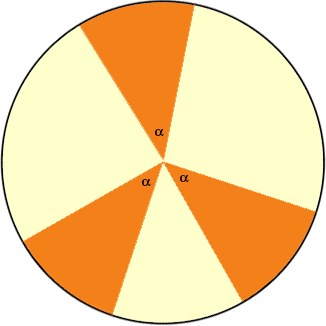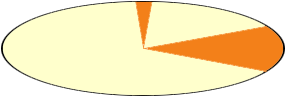Name: Wael Level: first secondary Who is asking: student How is the area of an arc (alpha*pi*r squared/360) derived? How is the length of an arc (alpha*pi*r/180) derived? Wael, The important factor that influences both of these expressions is the symmetry of the circle. If the central angle of a sector of a circle is alpha then the area of the sector is the same as the area of any other sector with central angle alpha, regardless of where the sector sits in the circle.The same is not true for other plane figures. For example in an ellipse two sectors with the same central angle can have quite different areas.The area of a circle of radius r isr2. If you have a sector with central angle 180o (half of 360o) then the area of the sector is half the area of the circle. That is  a sector with central angle 180o has area 180/360r2 = 1/2r2 If you have a sector with central angle 90o (one-quarter of 360o) then the area of the sector is one-quarter the area of the circle. That is  a sector with central angle 90o has area 90/360r2 = 1/4r2 If you have a sector with central angle 36o (one-tenth of 360o) then the area of the sector is one-tenth the area of the circle. That is  a sector with central angle 36o has area 36/360r2 = 1/10r2 The point I am making here is that the measure of the central angle is a fraction of 360o and the area of a sector is a fraction of the area of a circle, and these fractions are the same. So one more example, if the measure of the central angle iso (/360 of 360o) then the area of the sector is/360r2 A similar arguent is valid for the length of an arc. The measure of the central angle is a fraction of 360o and the length of an arc is a fraction of the circumference of the circle, and these fractions are the same. I hope this helps, Penny